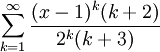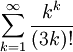# Quiz 2

Previous: Quiz 1

Next: Quiz 3

The following quiz covers topics in Unit 2.

If you would like to know how well you did on the quiz, you can have the quiz results sent to an email address that you provide at the end of the quiz. You will also be sent partial solutions.

## Quiz 2: Convergence Tests

Progress:

Consider the following infinite seriesDetermine, if possible, whether the infinite series converges.

Find all the values of x such that the following series will converge:Consider the infinite seriesThe divergence test

Consider the infinite seriesConsider the infinite seriesFor what values of p is the series convergent?

Previous: Quiz 1

Next: Quiz 3